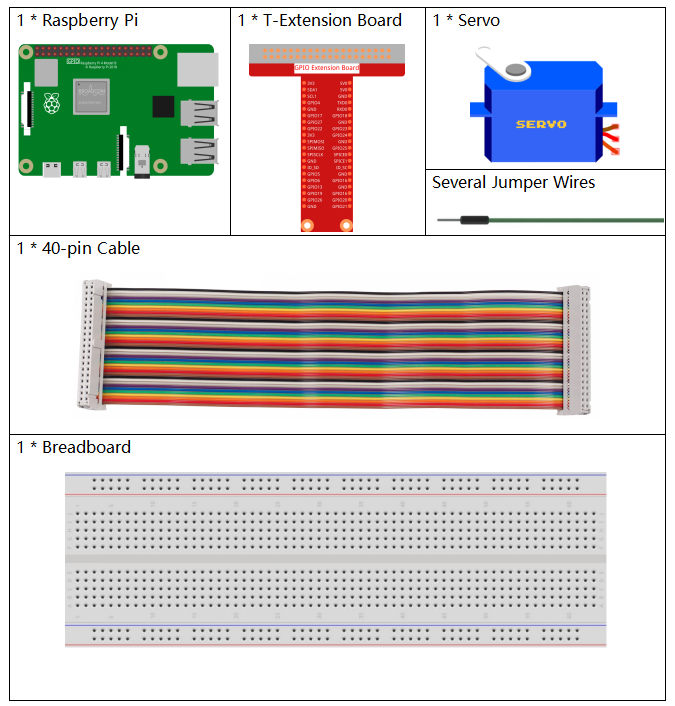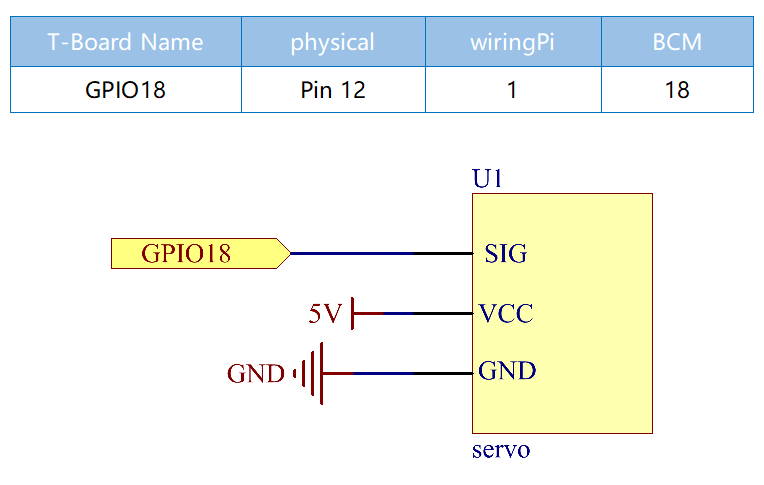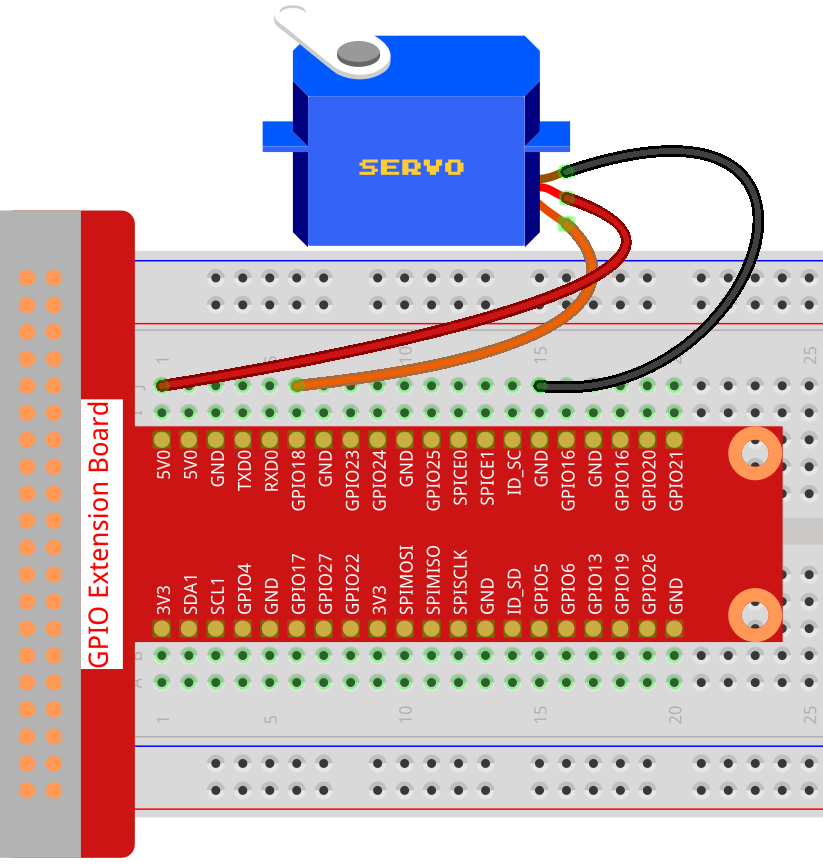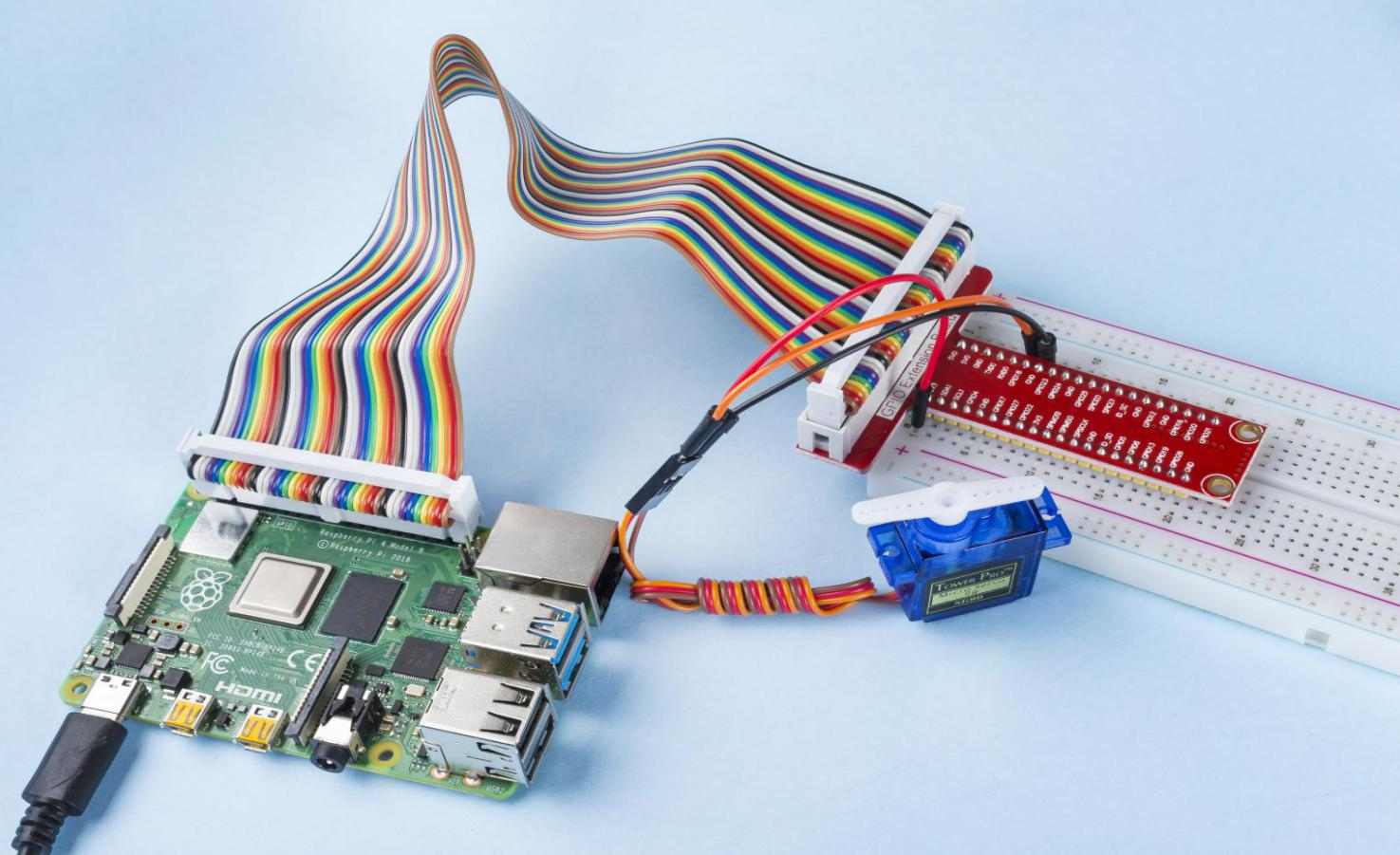# 1.3.2 Servo¶

## Introduction¶

In this project, we will learn how to make the servo rotate.

## Components¶## Schematic Diagram¶## Experimental Procedures¶

Step 1: Build the circuit.Step 2: Go to the folder of the code.

```cd /home/pi/raphael-kit/c/1.3.2
```

Step 3: Compile the code.

```gcc 1.3.2_Servo.c -lwiringPi
```

Step 4: Run the executable file.

```sudo ./a.out
```

After the program is executed, the servo will rotate from 0 degrees to 180 degrees, and then from 180 degrees to 0 degrees, circularly.

Note

If it does not work after running, or there is an error prompt: “wiringPi.h: No such file or directory”, please refer to C code is not working?.

Code

```#include <wiringPi.h>
#include <softPwm.h>
#include <stdio.h>

#define ServoPin    1       //define the servo to GPIO1
long Map(long value,long fromLow,long fromHigh,long toLow,long toHigh){
return (toHigh-toLow)*(value-fromLow) / (fromHigh-fromLow) + toLow;
}
void setAngle(int pin, int angle){    //Create a funtion to control the angle of the servo.
if(angle < 0)
angle = 0;
if(angle > 180)
angle = 180;
softPwmWrite(pin,Map(angle, 0, 180, 5, 25));
}

int main(void)
{
int i;
if(wiringPiSetup() == -1){ //when initialize wiring failed,print message to screen
printf("setup wiringPi failed !");
return 1;
}
softPwmCreate(ServoPin, 0, 200);       //initialize PMW pin of servo
while(1){
for(i=0;i<181;i++){     // Let servo rotate from 0 to 180.                  setAngle(ServoPin,i);
delay(2);
}
delay(1000);
for(i=181;i>-1;i--){        // Let servo rotate from 180 to 0.              setAngle(ServoPin,i);
delay(2);
}
delay(1000);
}
return 0;
}
```

Code Explanation

```long Map(long value,long fromLow,long fromHigh,long toLow,long toHigh){
return (toHigh-toLow)*(value-fromLow) / (fromHigh-fromLow) + toLow;
}
```

Create a `Map()` function to map value in the following code.

```void setAngle(int pin, int angle){    //Create a funtion to control the angle of the servo.
if(angle < 0)
angle = 0;
if(angle > 180)
angle = 180;
softPwmWrite(pin,Map(angle, 0, 180, 5, 25));
}
```

Create a funtion, `setAngle()` to write angle to the servo.

```softPwmWrite(pin,Map(angle,0,180,5,25));
```

This function can change the duty cycle of the PWM.

To make the servo rotate to 0 ~ 180 °, the pulse width should change within the range of 0.5ms ~ 2.5ms when the period is 20ms; in the function, `softPwmCreate()` , we have set that the period is 200x100us=20ms, thus we need to map 0 ~ 180 to 5x100us ~ 25x100us.

The prototype of this function is shown below.

```int softPwmCreate（int pin，int initialValue，int pwmRange）;
```
• `pin`: Any GPIO pin of Raspberry Pi can be set as PWM pin.

• `initialValue`: The initial pulse width is that initialValue times 100us.

• `pwmRange`: the period of PWM is that pwmRange times 100us.

## Phenomenon Picture¶